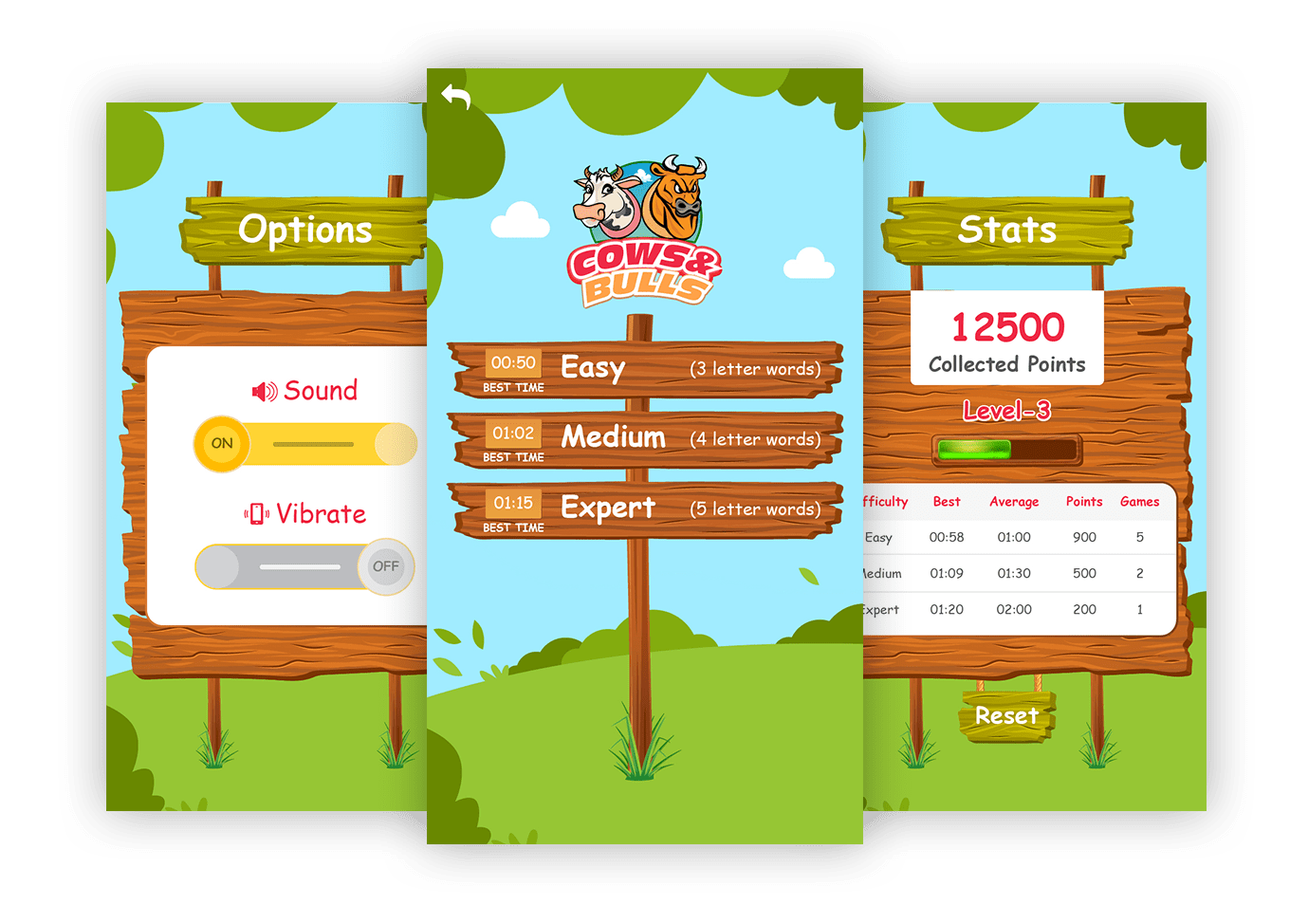Cows & Bulls is a classic game of guessing the secret word. It has 3-Letters, 4-Letters and 5-Letters version. The game is played by guessing a word and the opponent mentions the number of Cows (for the letters which are present in the secret word, but not in the guessed positions) and Bulls (for the letters which are present in the secret word, and also in the exact positions).

# Guessed

## Play GameFor example, if the secret word is GATE, and the first guessed word is SOIL would result in "0 Cows, 0 Bulls" (since all the guessed letters are not in the secret word). If the guessed word is TRIM it would result in "1 Cows, 0 Bulls" (since T is present, but not in the exact position), and if the guessed word is RAGE it would result in "1 Cows, 2 Bulls" (since A and E are in the right positions, while G is in the wrong position). The game would continue until you guess the right word with "4 Bulls".• A
• B
• C
• D
• E
• F
• G
• H
• I
• J
• K
• L
• M
• N
• O
• P
• Q
• R
• S
• T
• U
• V
• W
• X
• Y
• Z
• A
• B
• C
• D
• E
• F
• G
• H
• I
• J
• K
• L
• M
• N
• O
• P
• Q
• R
• S
• T
• U
• V
• W
• X
• Y
• Z

• #ee2945
• #ffa248
• #0087cb
• #ffd433
Back